# NCERT Exemplar Class 9 Science Solutions for Chapter 9 - Force And Laws Of Motion

## NCERT Exemplar Solutions Class 9 Science Chapter 9 – Free PDF Download

NCERT Exemplar solution for Class 9 Science Chapter 9 Force and Laws of Motion is provided here to assist the CBSE Class 9 students in comprehending the concept clearly and to clear all their doubts that arise while studying the concepts in the chapter. Solving Exemplar question help you to gain extra knowledge on all important Force and Laws of Motion chapter. Students can utilise these solutions to learn the correct answers for the questions present in their prescribed CBSE textbook. All the solutions created are very accurate and based on the latest CBSE Syllabus and guidelines.

This NCERT Exemplar will provide you answers to the questions given in the NCERT Exemplar book. There are 8 MCQS, 9 short answer questions and 3 long answer questions. Getting well versed with these questions will help you to get knowledge of the types of questions that appear and helps students practise before facing the main examination. Importantly, students can use the NCERT Exemplar Solutions for Class 9 PDF for revision purposes as it contains all the main topics being highlighted.

### Download PDF of NCERT exemplar for Class 9 Science Chapter 9 – Force and Laws of Motion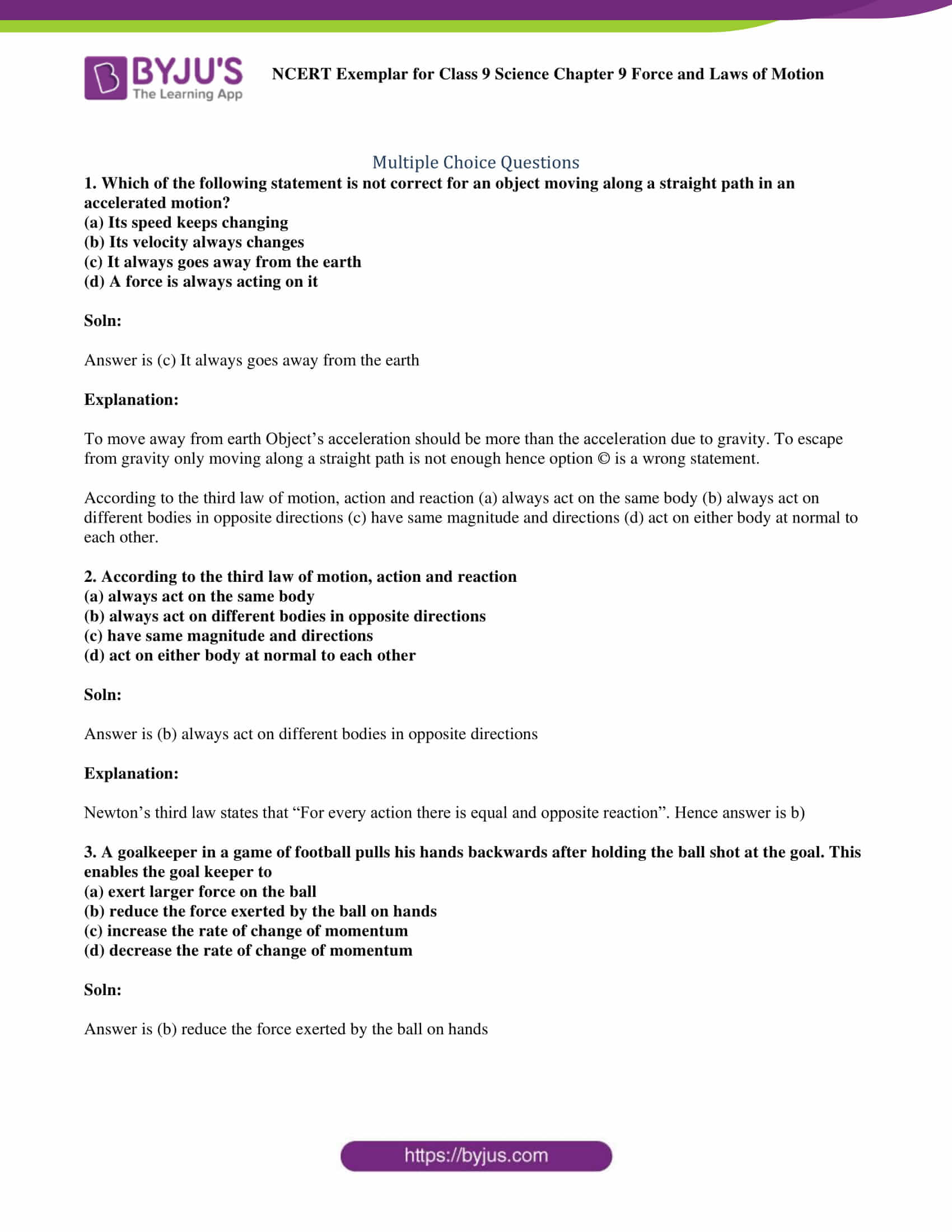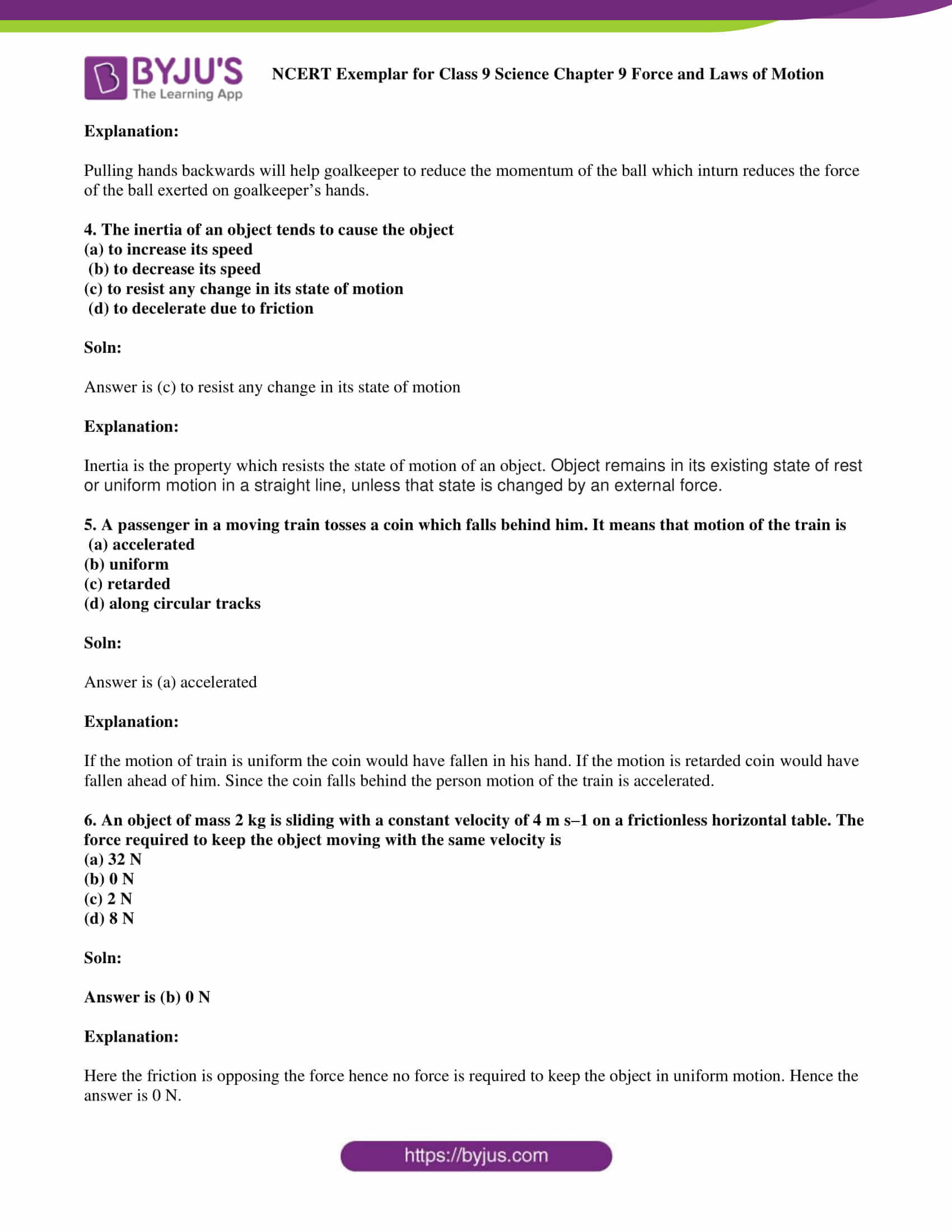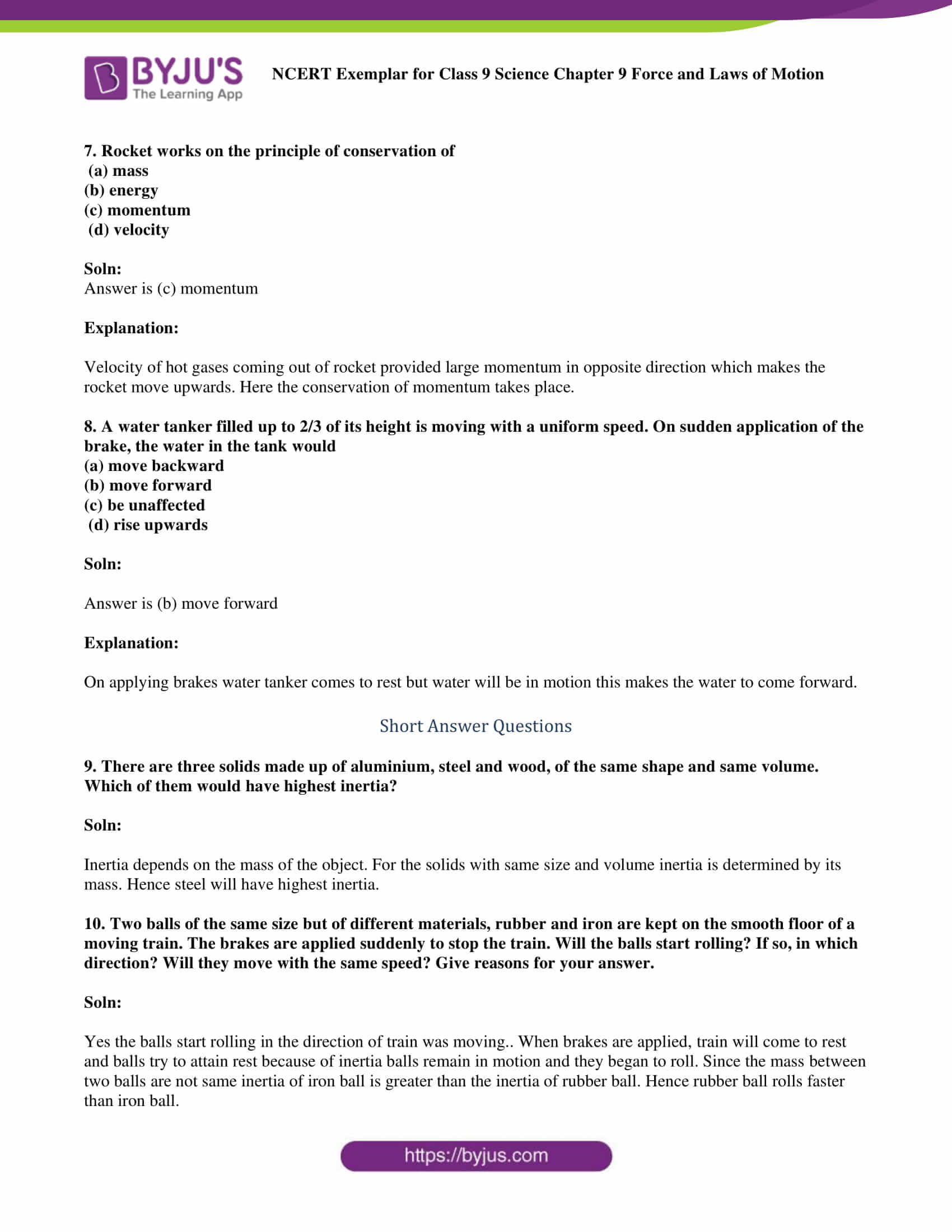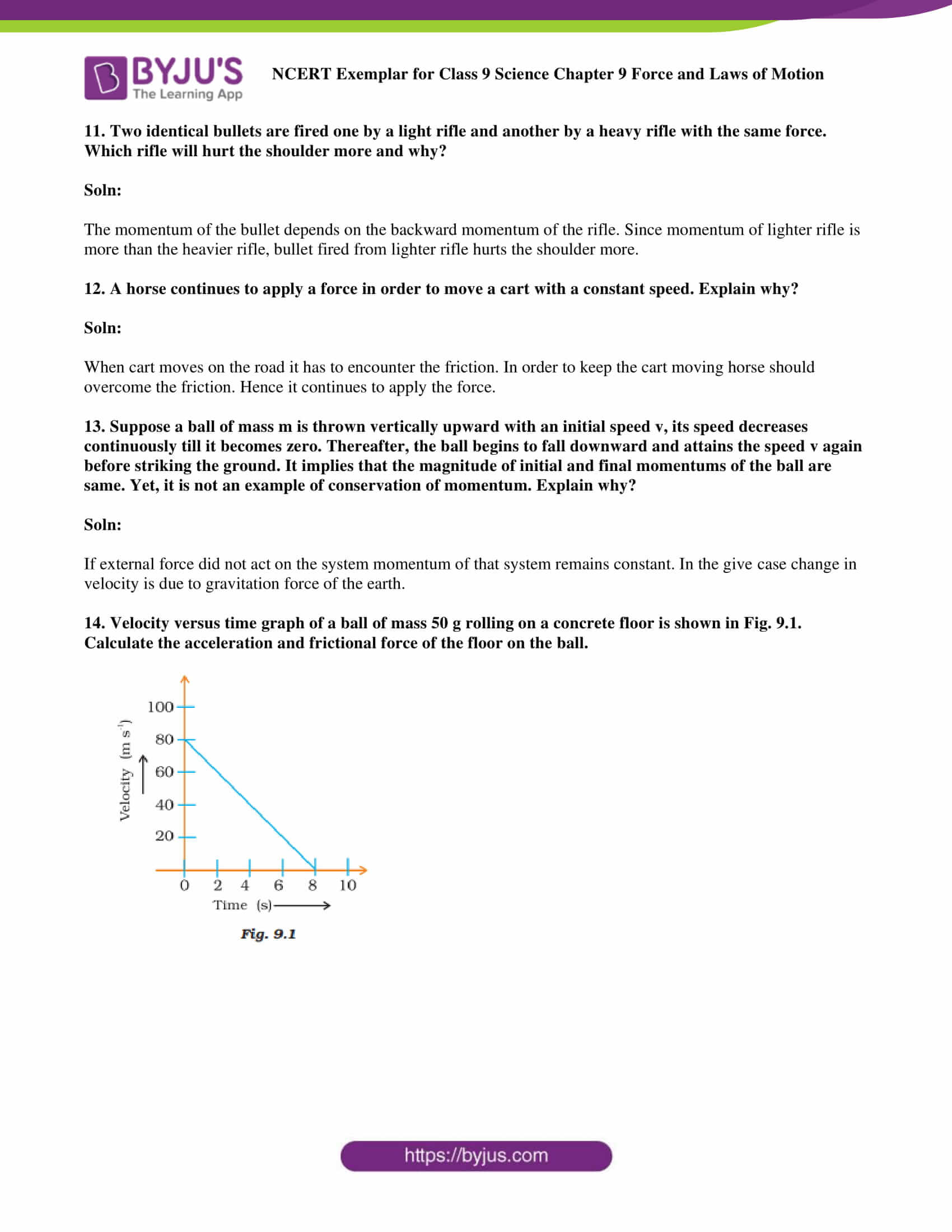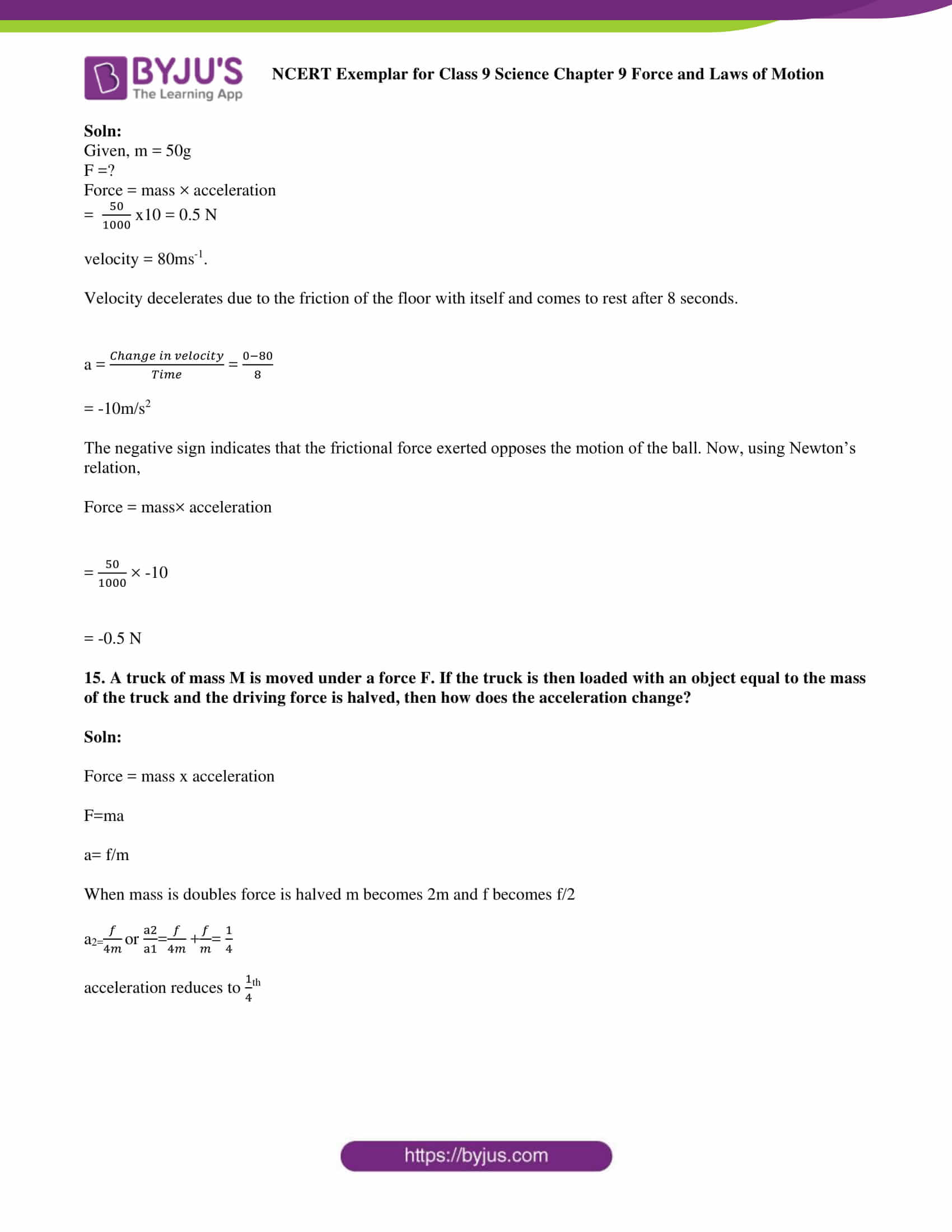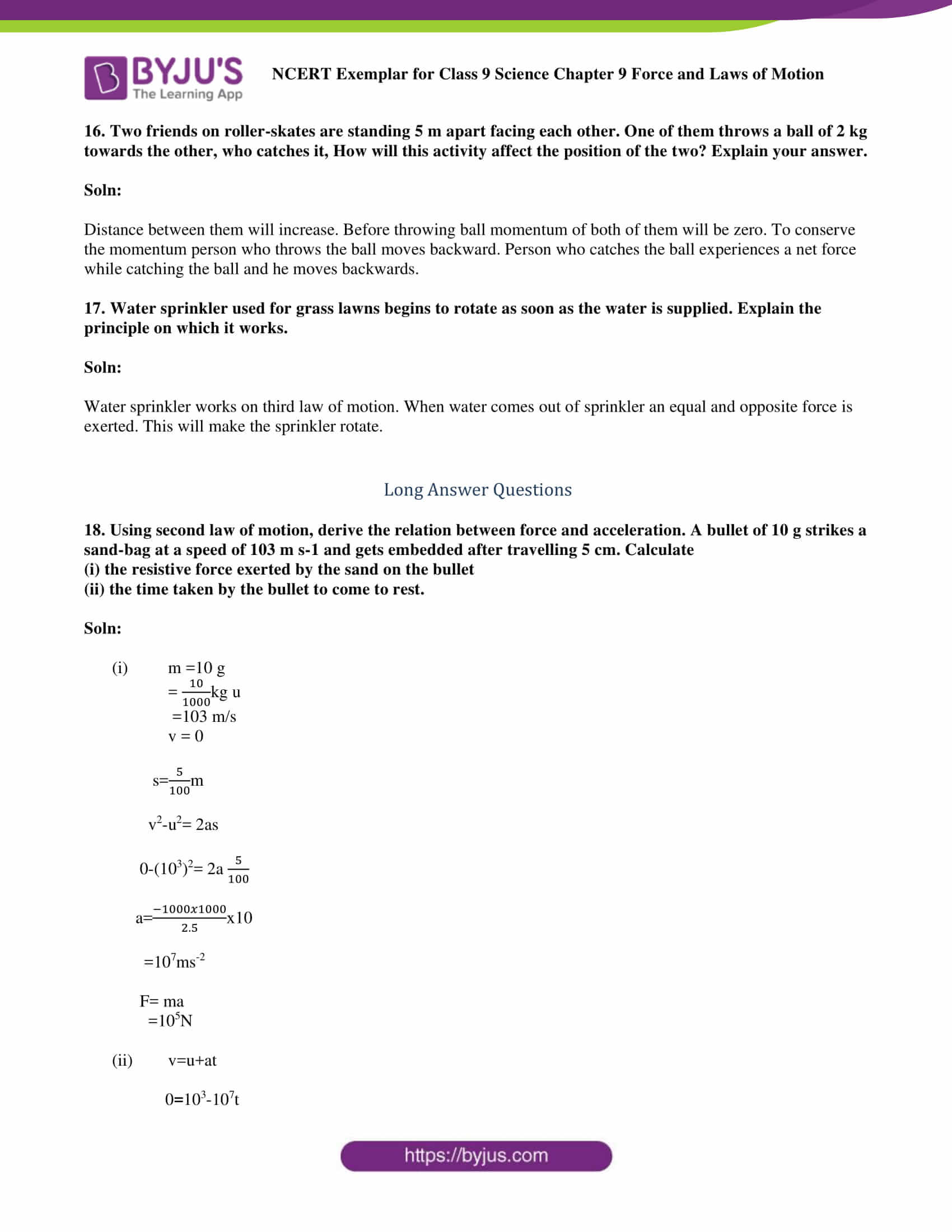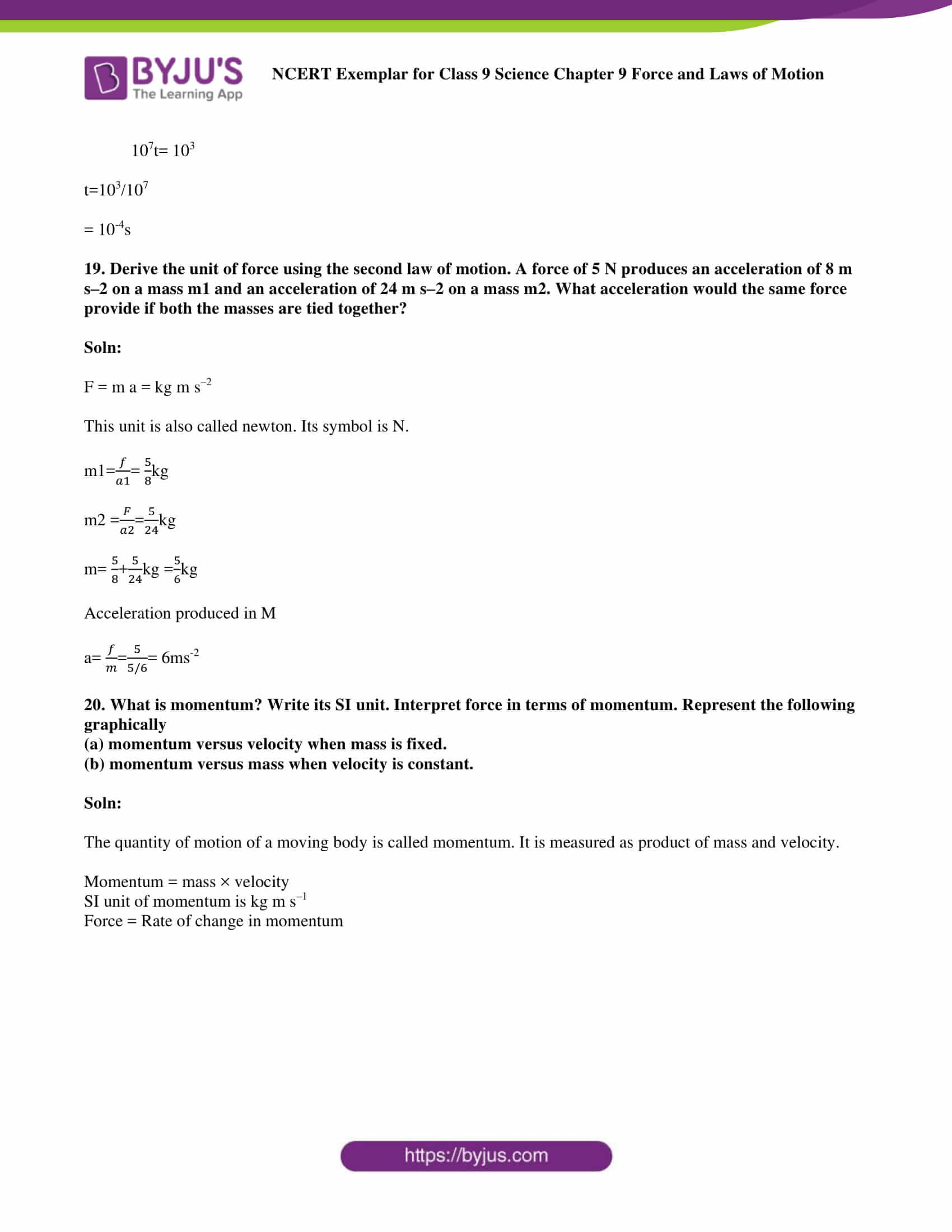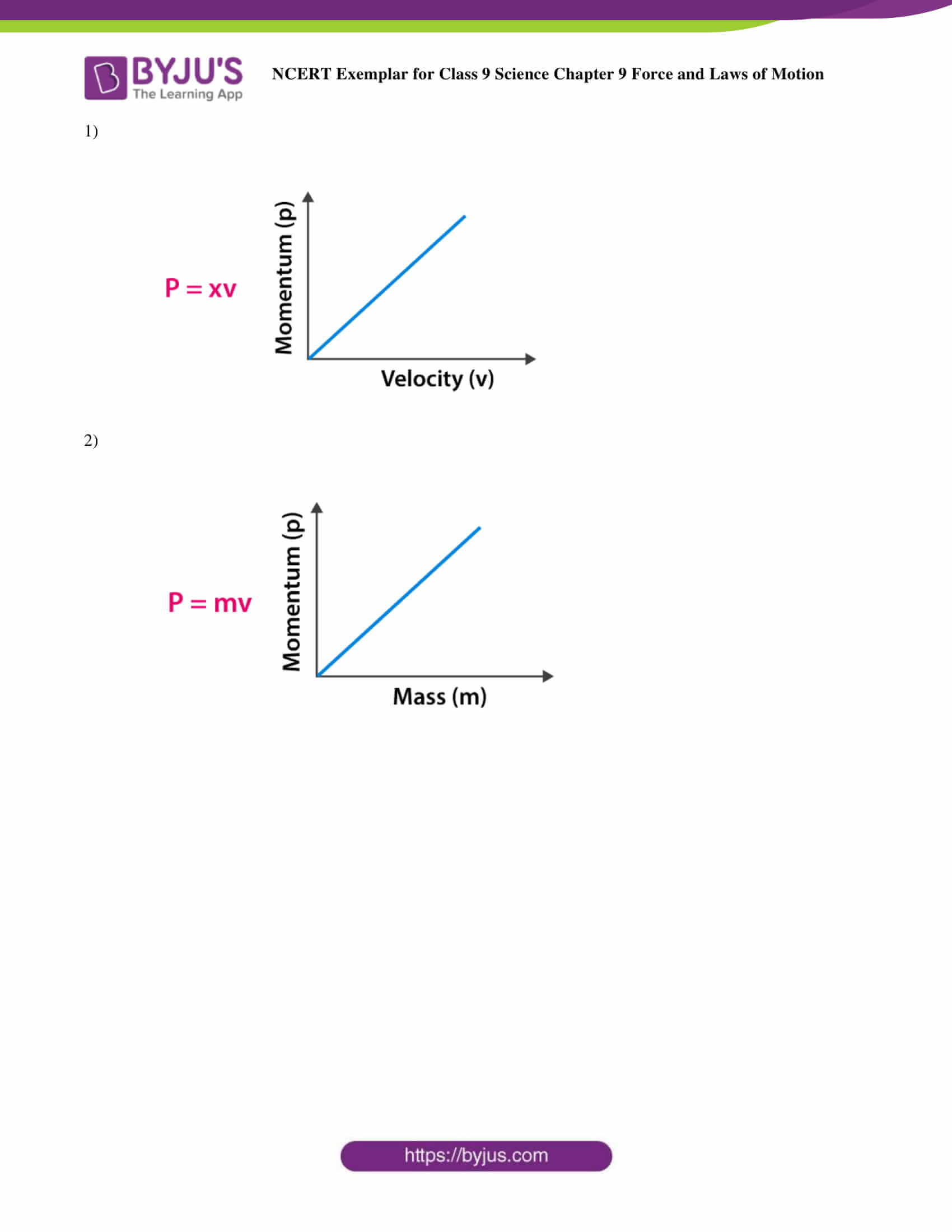### Multiple Choice Questions

1. Which of the following statement is not correct for an object moving along a straight path in an accelerated motion?

(a) Its speed keeps changing

(b) Its velocity always changes

(c) It always goes away from the earth

(d) A force is always acting on it

Soln:

Answer is (c) It always goes away from the earth

Explanation:

To move away from earth Object’s acceleration should be more than the acceleration due to gravity. To escape from gravity only moving along a straight path is not enough hence option (c) is a wrong statement.

According to the third law of motion, action and reaction (a) always act on the same body (b) always act on different bodies in opposite directions (c) have the same magnitude and directions (d) act on either body at normal to each other.

2. According to the third law of motion, action and reaction

(a) always act on the same body

(b) always act on different bodies in opposite directions

(c) have same magnitude and directions

(d) act on either body at normal to each other

Soln:

Answer is (b) always act on different bodies in opposite directions

Explanation:

Newton’s third law states that “For every action, there is an equal and opposite reaction”. Hence answer is b)

3. A goalkeeper in a game of football pulls his hands backwards after holding the ball shot at the goal. This enables the goal keeper to

(a) exert larger force on the ball

(b) reduce the force exerted by the ball on hands

(c) increase the rate of change of momentum

(d) decrease the rate of change of momentum

Soln:

Answer is (b) reduce the force exerted by the ball on hands

Explanation:

Pulling hands backwards will help goalkeeper to reduce the momentum of the ball which in turn reduces the force of the ball exerted on goalkeeper’s hands.

4. The inertia of an object tends to cause the object

(a) to increase its speed

(b) to decrease its speed

(c) to resist any change in its state of motion

(d) to decelerate due to friction

Soln:

Answer is (c) to resist any change in its state of motion

Explanation:

Inertia is the property which resists the state of motion of an object. The object remains in its existing state of rest or uniform motion in a straight line unless that state is changed by an external force.

5. A passenger in a moving train tosses a coin which falls behind him. It means that motion of the train is

(a) accelerated

(b) uniform

(c) retarded

(d) along circular tracks

Soln:

Explanation:

If the motion of the train is uniform the coin would have fallen in his hand. If the motion is retarded coin would have fallen ahead of him. Since the coin falls behind the person motion of the train is accelerated.

6. An object of mass 2 kg is sliding with a constant velocity of 4 m/s on a frictionless horizontal table. The force required to keep the object moving with the same velocity is

(a) 32 N

(b) 0 N

(c) 2 N

(d) 8 N

Soln:

Explanation:

Here the friction is opposing the force hence no force is required to keep the object in uniform motion. Hence, the answer is 0 N.

7. Rocket works on the principle of conservation of

(a) mass

(b) energy

(c) momentum

(d) velocity

Soln:

Explanation:

The velocity of hot gases coming out of the rocket provided large momentum in the opposite direction which makes the rocket move upwards. Here the conservation of momentum takes place.

8. A water tanker filled up to 2/3 of its height is moving with a uniform speed. On sudden application of the brake, the water in the tank would

(a) move backward

(b) move forward

(c) be unaffected

(d) rise upwards

Soln:

Explanation:

On applying brakes water tanker comes to rest but water will be in motion this makes the water to come forward.

9. There are three solids made up of aluminium, steel and wood, of the same shape and same volume. Which of them would have highest inertia?

Soln:

Inertia depends on the mass of the object. For the solids with the same size and volume, inertia is determined by its mass. Hence steel will have the highest inertia.

10. Two balls of the same size but of different materials, rubber and iron are kept on the smooth floor of a moving train. The brakes are applied suddenly to stop the train. Will the balls start rolling? If so, in which direction? Will they move with the same speed? Give reasons for your answer.

Soln:

Yes, the balls start rolling in the direction of the train’s movement. When brakes are applied, the train will come to rest and balls try to attain rest because of inertia balls remain in motion and they begin to roll. Since the mass between two balls is not the same, the inertia of the iron ball is greater than the inertia of the rubber ball. Hence, rubber ball rolls faster than the iron ball.

11. Two identical bullets are fired one by a light rifle and another by a heavy rifle with the same force. Which rifle will hurt the shoulder more and why?

Soln:

The momentum of the bullet depends on the backward momentum of the rifle. As per Newton’s third law of motion: recoil velocity is equivalent to the bullet’s velocity, making it lighter. Since the momentum of the lighter rifle is more than the heavier rifle, the bullet fired from lighter rifle hurts the shoulder more.

12. A horse continues to apply a force in order to move a cart with a constant speed. Explain why?

Soln:

When cart moves on the road it has to encounter friction. In order to keep the cart moving horse should overcome the friction. Hence it continues to apply the force.

13. Suppose a ball of mass m is thrown vertically upward with an initial speed v, its speed decreases continuously till it becomes zero. Thereafter, the ball begins to fall downward and attains the speed v again before striking the ground. It implies that the magnitude of initial and final momentums of the ball are same. Yet, it is not an example of conservation of momentum. Explain why?

Soln:

If the external force did not act on the system, the momentum of that system remains constant. In the given case, change in velocity is due to the gravitational force of the earth and hence is not an example of conservation of momentum.

14. Velocity versus time graph of a ball of mass 50 g rolling on a concrete floor is shown in Fig. 9.1. Calculate the acceleration and frictional force of the floor on the ball.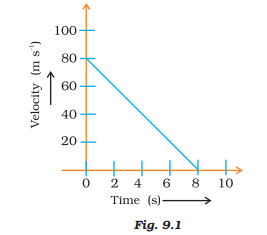Soln:

Given, m = 50g

F =?

Force = mass × acceleration

$$\begin{array}{l}\frac{50}{100}\end{array}$$
x 10 = 0.5 N

velocity = 80ms-1

Velocity decelerates due to the friction of the floor with itself and comes to rest after 8 seconds.

a =

$$\begin{array}{l}\frac{change in velocity}{time}\end{array}$$
=
$$\begin{array}{l}\frac{0-80}{s}\end{array}$$

= -10m/s2

The negative sign indicates that the frictional force exerted opposes the motion of the ball. Now, using Newton’s relation,

Force = mass× acceleration

$$\begin{array}{l}\frac{50}{1000}\end{array}$$
× -10

= -0.5 N

15. A truck of mass M is moved under a force F. If the truck is then loaded with an object equal to the mass of the truck and the driving force is halved, then how does the acceleration change?

Soln:

Force = mass x acceleration

F=ma

a= f/m

When mass is doubled force is halved m becomes 2m and f becomes f/2

a2=f/4m or a2/a1 = f/4m + f/4m = 1/4

acceleration reduces to

$$\begin{array}{l}\frac{1}{4}\end{array}$$
th

16. Two friends on roller-skates are standing 5 m apart facing each other. One of them throws a ball of 2 kg towards the other, who catches it, How will this activity affect the position of the two? Explain your answer.

Soln:

Distance between them will increase. Before throwing the ball, the momentum of both of them will be zero. To conserve the momentum, the person who throws the ball moves backwards. The person who catches the ball experiences a net force while catching the ball and he moves backwards.

17. Water sprinkler used for grass lawns begins to rotate as soon as the water is supplied. Explain the principle on which it works.

Soln:

Water sprinkler works on the third law of motion. When water comes out of sprinkler an equal and opposite force is exerted. This will cause the sprinkler to rotate.

18. Using second law of motion, derive the relation between force and acceleration. A bullet of 10 g strikes a sand-bag at a speed of 103 m/s and gets embedded after travelling 5 cm. Calculate

(i) the resistive force exerted by the sand on the bullet

(ii) the time taken by the bullet to come to rest.

Soln:

(i) m =10 g

=

$$\begin{array}{l}\frac{10}{1000}\end{array}$$
kg u

= 103 m/s

v = 0

s=

$$\begin{array}{l}\frac{5}{100}\end{array}$$
m

v2-u2= 2as

0-(103)2= 2a

$$\begin{array}{l}\frac{5}{100}\end{array}$$

a =

$$\begin{array}{l}\frac{-1000 × 1000}{2.5}\end{array}$$
x 10

=107ms-2

F = ma

=105N

(ii) v=u+at

0=103-107t

107t= 103

t=103/107

= 10-4s

19. Derive the unit of force using the second law of motion. A force of 5 N produces an acceleration of 8 ms-2 on a mass m1 and an acceleration of 24 ms-2 on a mass m2. What acceleration would the same force provide if both the masses are tied together?

Soln:

F = m a = kg m s–2

This unit is also called newton. Its symbol is N.

m1=

$$\begin{array}{l}\frac{f}{a1}\end{array}$$
=
$$\begin{array}{l}\frac{5}{8}\end{array}$$
kg

m2 =

$$\begin{array}{l}\frac{f}{a2}\end{array}$$
=
$$\begin{array}{l}\frac{5}{24}\end{array}$$
kg

m=

$$\begin{array}{l}\frac{5}{8}\end{array}$$
+
$$\begin{array}{l}\frac{5}{24}\end{array}$$
kg

=

$$\begin{array}{l}\frac{5}{6}\end{array}$$
kg

Acceleration produced in M

a=

$$\begin{array}{l}\frac{f}{m}\end{array}$$
=
$$\begin{array}{l}\frac{5}{5/6}\end{array}$$
= 6ms-2

20. What is momentum? Write its SI unit. Interpret force in terms of momentum. Represent the following graphically

(a) momentum versus velocity when mass is fixed.

(b) momentum versus mass when velocity is constant.

Soln:

The quantity of motion of a moving body is called momentum. It is measured as product of mass and velocity.

Momentum = mass × velocity

SI unit of momentum is kg m s–1

Force = Rate of change in momentum

1)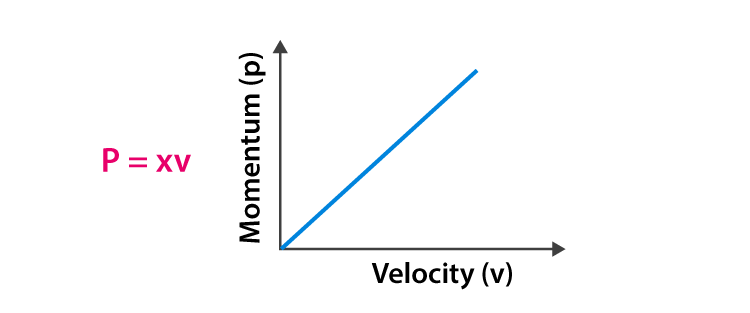2)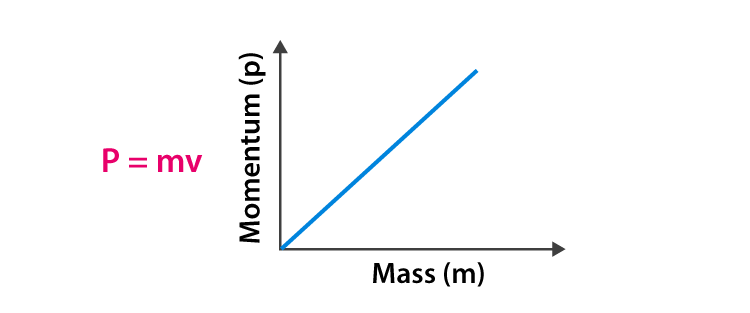### Topics included in Class 9 Science Chapter 9 Exemplar Force and Laws of Motion

1. Balanced And Unbalanced Forces
2. First Law Of Motion
3. Inertia And Mass
4. Second Law Of Motion
5. Mathematical Formulation Of Second Law Of Motion
6. Third Law Of Motion
7. Conservation Of Momentum.

Learning the Force and Laws of Motion is very much necessary to understand the advanced topics in higher classes. After reading the chapter students will learn about the conservation of momentum, the three laws of motion, inertia, mass, and friction. Solve higher order thinking questions from this chapter by solving the NCERT Exemplar Science Class 9 Chapter 9 Force and Laws of Motion.

BYJU’S innovative teaching methodology helps you in understanding the concepts easily. This will make your study easier and aids you in acing the examination. Our study materials help you comprehend the topic clearly. BYJU’S teachers also provide you with periodic insights on your progress through assignments and exercises. To avail all the benefits, login to the BYJU’S website or download BYJU’S learning App.

## Frequently Asked Questions on NCERT Exemplar Solutions for Class 9 Science Chapter 9

### What is Newton’s first law of motion in the Chapter 9 of NCERT Solutions for Class 10 Science?

Newton’s first law of motion implies that things cannot start, stop, or change direction all by themselves, and it requires some force from the outside to cause such a change. This property of massive bodies to resist changes in their state of motion is called inertia. The first law of motion is also known as the law of inertia. Students can refer to the Chapter 9 of NCERT Exemplar Solutions for Class 9 Science while answering the textbook questions to get a clear idea of the concepts. Both chapter wise and exercise wise solutions are available which can be used by the students based on their needs.

### What are the topics covered in the Chapter 9 of NCERT Exemplar Solutions for Class 9 Science?

The topics covered in the Chapter 9 of NCERT Exemplar Solutions for Class 9 Science are –
1. Balanced And Unbalanced Forces
2. First Law Of Motion
3. Inertia And Mass
4. Second Law Of Motion
5. Mathematical Formulation Of Second Law Of Motion
6. Third Law Of Motion
7. Conservation Of Momentum.

### Does the NCERT Exemplar Solutions for Class 9 Science Chapter 9 help students to score well in the board exams?

The solutions are elaborate and detailed to help students understand the concepts effortlessly. The faculty at BYJU’S frame the solutions to meet the CBSE syllabus and guidelines. Simple and easy to understand language is used to help students grasp the method of answering complex questions easily. Sample papers and previous year question papers are also solved with the aim of improving analytical and logical thinking abilities of students.